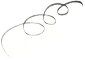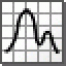by PTC Inc

## Engineering software suitable for Windows

Operating system: Windows

Publisher: PTC Inc

Antivirus check: passed

Last revision: Last week

Report a Problem

MathCAD is an advanced specialized software solution that allows its users to make mathematical and technical calculations of various complexity. At first glance, the application's interface seems unusual and confusing, but in an hour or two you'll realize how simple and intuitive it is. MathCAD includes several toolbars that allow you to work with formulas and tables. In addition, the application supports more than a hundred operators and logical functions, and allows you to unleash tasks that have both numerical and symbolic solutions. If you consider the above described functionality to be insufficient for comfortable work, you can download additional modules for the program from the official website of the developer at any time. In general, MathCAD is a great helper for technical professions.

- has an extremely simple and intuitive interface;

- can be extended with additional modules;

- allows you to find symbolic and numerical solutions;

- copes with the challenges of any complexity;

- supports more than a hundred operators.

Archie

Mathcad is a engineering math software calculation application tool.mathcad application only runs in microsoft windows.it will improve the accuracy and time to market through atomatic unit convertion. you can able to quickly modify calculation to drive and the geometry of the design. you can access calculations and documentation.it is easy to use.if you use you will just love it!.it is a safe application tool.
Jake

Mathcad for windows is used for Math calculation in engineering Maths and all maths. its encouraging in all maths. So mathcad is very used in calculation of all. in this software is very used for engineering maths and maths. So mathcad is very easy and handling is perfect and answer is suitable on maths. So mathcad for windows is using in calculation and maths subject orinted only. So installation is easy.
Logan H*****j

Mathcad is a powerful and easy-to-use software program for engineering and scientific calculations. It is a computer algebra system that enables users to easily create, solve, and visualize mathematical equations. Mathcad is used by engineers, scientists, and mathematicians to solve complex problems in a variety of industries. It can be used to solve problems involving equations, numerical integration, linear and non-linear optimization, and more. Mathcad also offers interactive graphing and plotting capabilities, allowing users to quickly visualize the results of their calculations.
Aaron C******w

Mathcad is a powerful piece of engineering software. It offers a wide range of mathematical calculations and visualizations. The user interface is intuitive and the results are reliable and accurate. I have used Mathcad extensively to analyze complex engineering problems. The built-in library of mathematical functions makes it easy to design and test calculations. Mathcad also provides excellent graphing capabilities, allowing me to quickly visualize the results of my calculations. In addition, it can be easily integrated with other software, allowing me to use Mathcad as an engineering tool. Overall, I found Mathcad to be a great tool for engineering calculations and visualization.
Andrew F******u

Mathcad sfotware is really usfeul for solving complex math problems, but it can be a bit difficukt to learn at the beginning.
Rory G.

Mathematical calculations and visualizations are easily performed with this software, making complex problems simpler to solve. The interface is user-friendly and the documentation is comprehensive. The software is well-suited for technical and scientific applications, but may not be necessary for everyday use. Some users may find the price point to be prohibitive.
Oliver Denais

Mathcad software is a powerful tool for performing mathematical calculations and analyzing data.